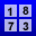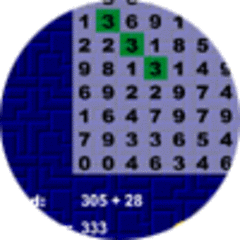Search
SoftwareThis is a traditional word search game with a mathematical twist. Instead of searching for words, you search for numbers representing the answers to an equation. You need to be able to calculate the answer to the equation and then search the answer in a grid of numbers.